## 6.13Turbulent kinetic energy

The Reynolds stress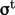in the ensemble-averaged momentum conservation Eq. (6.15 ) can be decomposed into viscous and pressure components. The turbulent pressure term,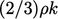, is commonly subsumed within pressure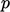, to give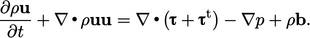(6.25)
The viscous stresses can be combined assuming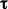is modelled as a Newtonian ﬂuid Eq. (2.41 ) and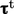by the eddy viscosity model Eq. (6.20 ) to give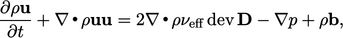(6.26)
where the eﬀective kinematic viscosity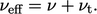(6.27)
The eﬀective viscosity represents momentum diﬀusion from the combined molecular and turbulent motions. The properties due to molecular motion are often described as laminar, e.g. laminar viscosity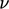.

Averaging the momentum equation and introducing the eddy viscosity model creates one additional unknown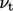. Additional models are required forto close the system of equations.

By considering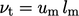from Sec. 6.11 , the model foris typically decomposed into components representing velocity and length scales,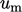and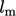respectively.

The scale of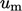corresponds to turbulent ﬂuctuations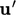, so it is reasonable to assume that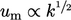. Since the ﬁeld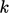is representative of the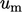component of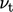, it is commonly adopted within turbulence models based on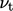. Also, it is well captured by a suitable conservation equation.

### Transport of turbulent kinetic energy

Conservation of turbulent kinetic energycan be written as: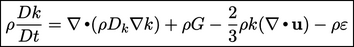(6.28)
where the turbulence generation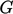is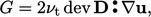(6.29)
and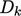is the eﬀective diﬀusivity for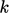. The equation is derived in a manner similar to Eq. (2.56 ) for conservation of speciﬁc internal energy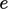, by (ensemble) averaging the separate energy contributions from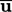and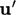. Whileincludes the kinetic energy of molecular motion,is the equivalent for eddy motion.

In Eq. (2.56 ), energy from bulk motion is passed to the submicroscopic scale as heat by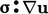. In Eq. (6.28), it is converted to turbulent energy by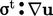using Boussinesq’s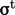from Eq. (6.20 ). The shear component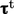provides the non-recoverable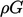in Eq. (6.28) and the second (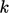) term yields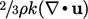.

Whiletransfers kinetic energy from the bulk ﬂow to, the ﬁnal term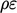transferson to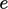as dissipated heat. Here,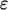is the turbulent dissipation rate per unit mass from in Sec. 6.6 which, from an ensemble-averaged derivation of Eq. (6.28), is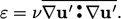(6.30)
Finally, diﬀusion of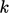is represented by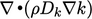, where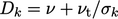. This represents diﬀusion by both molecular and turbulent motions and interactions, including an adjustable coeﬃcient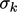which is usually set to 1.

Theequation goes part of the way to closing our system of equations. However, it introduces an additional unknown,, and the model for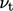still requires length scale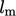.

Notes on CFD: General Principles - 6.13 Turbulent kinetic energy